# Writing Algebraic Expressions Lesson Plan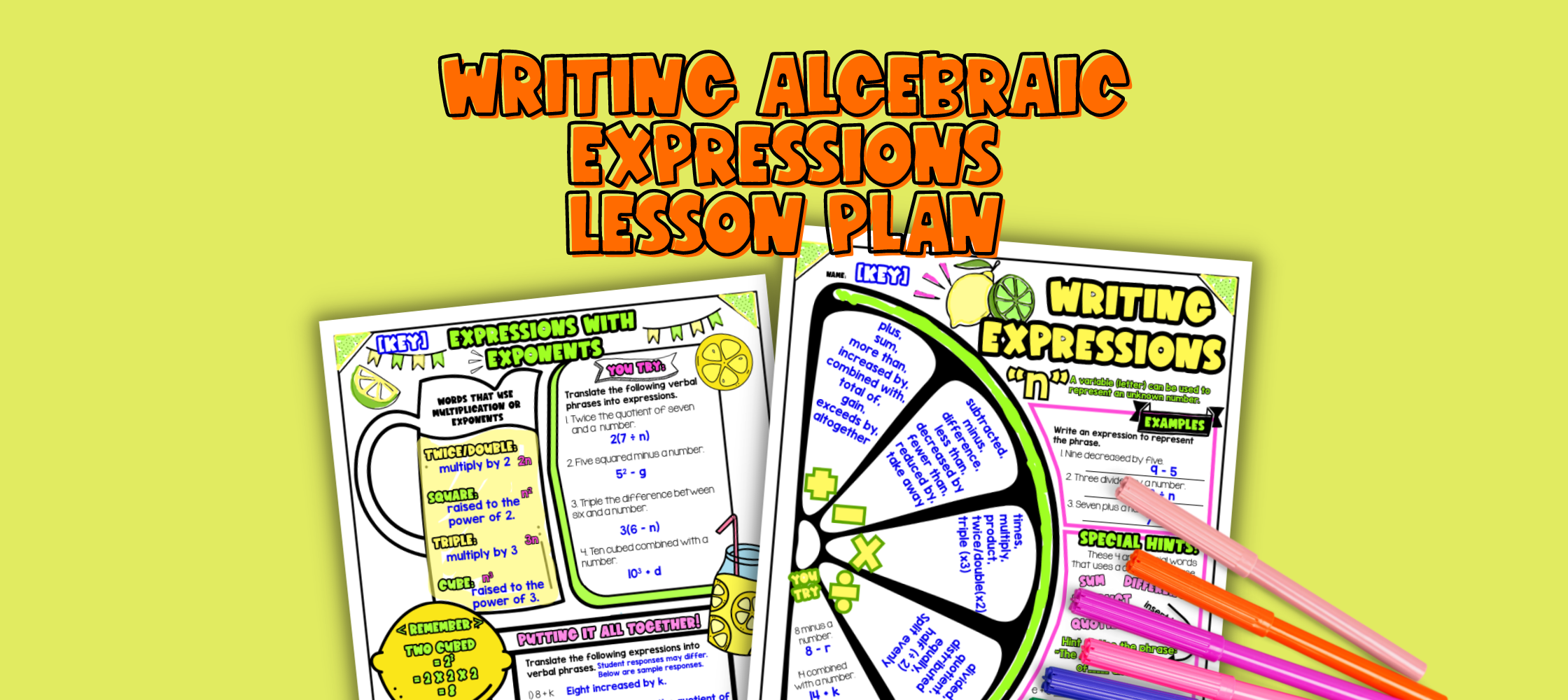Pin This

## Overview

Have you ever wondered how to teach your 6th or 7th grade students writing algebraic expressions from word problems?

In this lesson plan, students will learn how to write expressions, translate verbal phrases into algebraic expressions and work with expressions that include exponents. This artistic lesson plan includes guided notes (interactive sketch notes), check for understanding questions, and practice with a maze activity as well as a doodle and color by number activity to build understanding.

The lesson culminates with a real-life application where students learn how algebraic expressions help them to model a lemonade stand to make the most profit.

## Get the Lesson Materials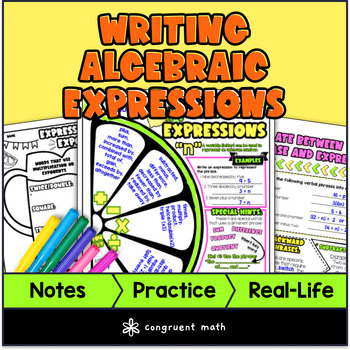\$4.25

## Learning Objectives

After this lesson, students will be able to:

• Write algebraic expressions to represent real-life situations

• Translate verbal phrases into algebraic expressions

• Work with expressions that include exponents

• Understand how algebraic expressions can be used in a real-world application (e.g. to model costs, revenue, and profit in a lemonade stand business)

## Prerequisites

Before this lesson, students should be familiar with:

• Basic math operations (addition, subtraction, multiplication, and division)

• Understanding of variables and their use in equations

• Basic understanding of exponents (optional, but helpful)

Materials:

## Key Vocabulary

• Algebraic expressions

• Sum, Difference, Product, Quotient (and more keywords specific to each operations!)

• Verbal phrases

• Exponents

• Costs, revenue, and profit

## Procedure

### Introduction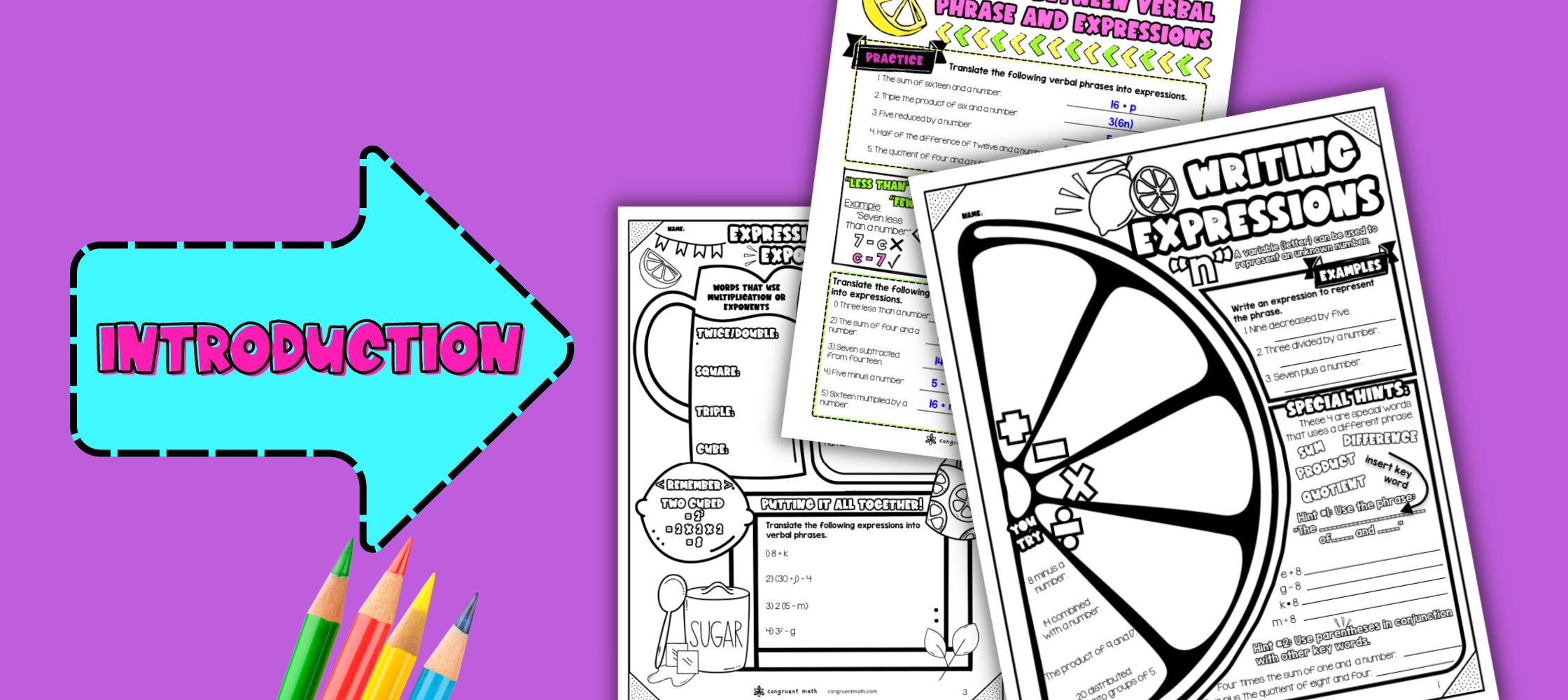Pin This
• As a hook, ask about whether they’ve ever started a business like a lemonade stand? What were the costs in the business, and how do they know if they’re making money? Refer to the last page of the guided notes as well as the FAQs below for ideas.

• Use the first page of the guided notes to introduce writing expressions, specifically key words associated with each of the four operations. Then have students practice translating verbal phrases to expressions and expressions back to verbal phrases. Refer to the FAQ below for ideas on how to respond to common student questions.

• Check for Understanding. Have students walk through the “You Try!” section. Call on students to talk through their answers. Based on student responses, reteach concepts that students need extra help with.

• Use the second page of the guided notes to introduce translating between verbal phrase and expressions. Refer to the FAQ below for ideas on how to respond to common student questions.

• Check for Understanding. Have students walk through the “Practice” section. Call on students to talk through their answers. Based on student responses, reteach concepts that students need extra help with.

• Use the guided notes to introduce expressions with exponents. Refer to the FAQ below for a walk through on this, as well as ideas on how to respond to common student questions.

• Check for Understanding. Have students walk through the “You Try!” and “Putting It all Together” sections. Call on students to talk through their answers, potentially on the whiteboard or projector. Based on student responses, reteach concepts that students need extra help with.

• If your class has a wide range of proficiency levels in the various checks for understanding, you can pull out students for reteaching, and have more advanced students begin work on the practice exercises.

### Practice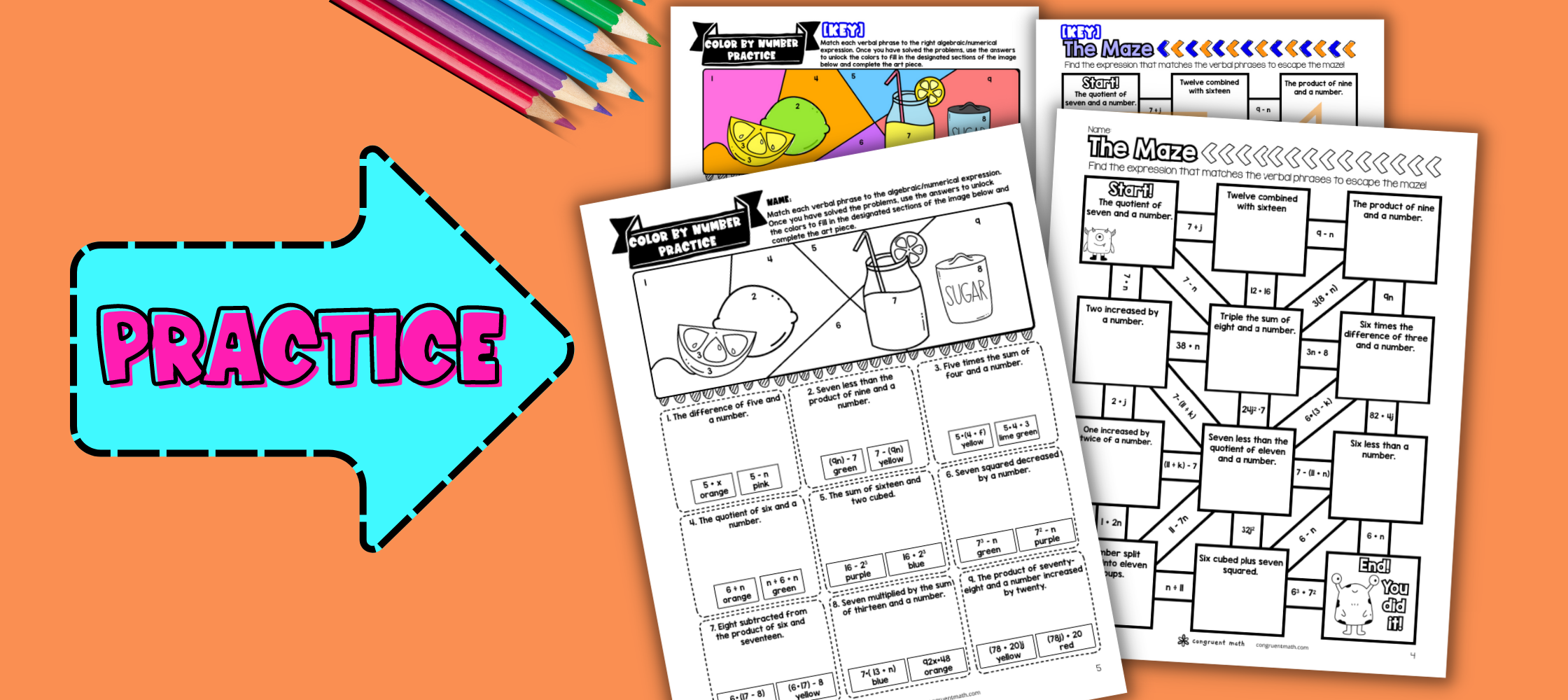Pin This
• Have students practice writing algebraic expressions from verbal phrases and key words using the maze activity included in the lesson plan. Walk around to answer student questions.

• Fast finishers can dive into the doodle math activity for extra practice. You can assign it as homework for the remainder of the class.

### Real-Life Application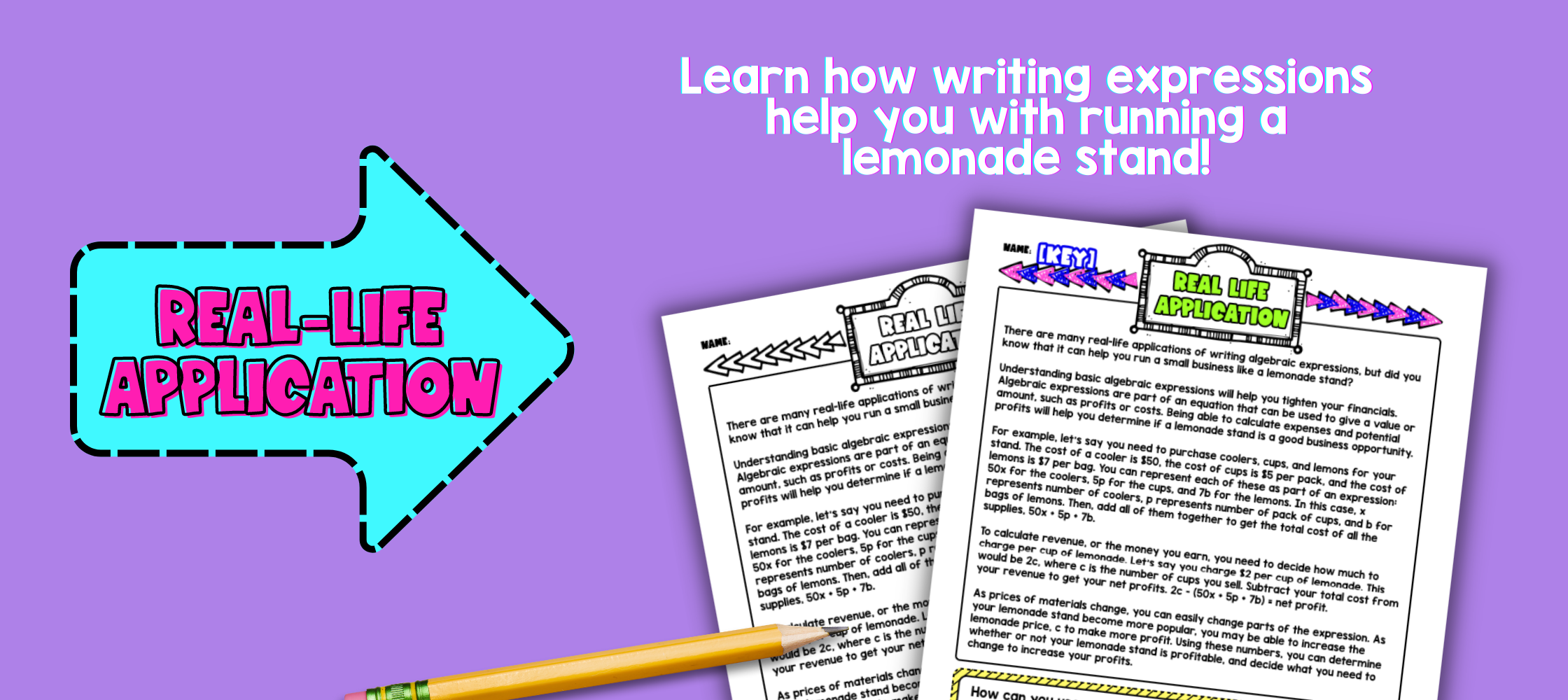Pin This
• Bring the class back together, and introduce the concept of running a lemonade stand as a real-life application that requires the use of algebraic expressions. Students will learn how to use algebraic expressions to model costs, revenue, and profit in a lemonade stand business. Refer to the FAQ for more ideas on how to teach it!